Learn data science and machine learning by building real-world projects on Jovian

## Classification of Images in Multi-class Weather Dataset (MWD) using Deep-Learning in PyTorch

This is my project for the course "Deep Learning with PyTorch: Zero to GANs" where I train a deep learning model in Pytorch from scratch for weather analysis by making it predict and recognize various weather patterns and conditions from still (colour)images.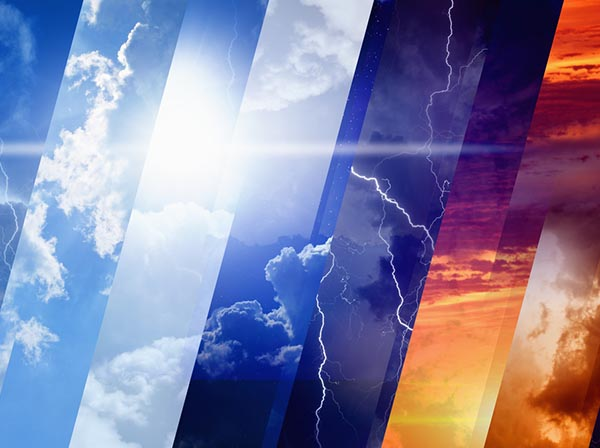PyTorch is an optimized open source tensor library for deep learning using GPUs and CPUs. It is based on the Torch library, used for applications such as computer vision and natural language processing, primarily developed by Facebook's AI Research lab. It is highly popular for its Automatic Differentiation feature and CUDA support.

Multi-class weather dataset(MWD) for image classification is a valuable dataset featured in the research paper entitled “Multi-class weather recognition from still image using heterogeneous ensemble method”. The dataset provides a platform for outdoor weather analysis by extracting various features for recognizing different weather conditions. This dataset is a collection of 1125 images divided into four groups ---> sunrise, shine, rain, and cloudy.

## Objective

To train a deep learning model in successfully recognizing various weather conditions while working reasonably well under constraints of computation by classifying images from the MWD using state-of-the-art techniques like using different CNNs (Convolutional Neural Network), Data Augmentation, Regularization, ResNet,etc and achieve a satisfactory accuracy.

Let's get started by importing some necessary libraries.

In :
``````import os
import torch
import torchvision
from zipfile import ZipFile
import torch.nn as nn
import numpy as np
import torch.nn.functional as F
from torchvision.datasets import ImageFolder
import torchvision.transforms as tt
from torch.utils.data import random_split
from torchvision.utils import make_grid
import matplotlib
import matplotlib.pyplot as plt``````

## Preparing and Exploring the Multi-Class Weather Dataset

First of all, we need to download the dataset and extract it.

In :
``````# Download the dataset
!wget https://www.dropbox.com/s/4g82t3edrdzbjpr/multi-class-weather-dataset.zip?dl=0

# Extract from archive
!mv multi-class-weather-dataset.zip?dl=0 multi-class-weather-dataset.zip
ZipFile("multi-class-weather-dataset.zip").extractall("/content/data")  ``````
```--2021-01-09 18:17:31-- https://www.dropbox.com/s/4g82t3edrdzbjpr/multi-class-weather-dataset.zip?dl=0 Resolving www.dropbox.com (www.dropbox.com)... 162.125.3.18, 2620:100:6018:18::a27d:312 Connecting to www.dropbox.com (www.dropbox.com)|162.125.3.18|:443... connected. HTTP request sent, awaiting response... 301 Moved Permanently Location: /s/raw/4g82t3edrdzbjpr/multi-class-weather-dataset.zip [following] --2021-01-09 18:17:31-- https://www.dropbox.com/s/raw/4g82t3edrdzbjpr/multi-class-weather-dataset.zip Reusing existing connection to www.dropbox.com:443. HTTP request sent, awaiting response... 302 Found Location: https://uc22f530c98ccbdd1ffd358dbd47.dl.dropboxusercontent.com/cd/0/inline/BGu9exMtBR-4l50l1UMwCa9r14h4DHTLf2k1P4RKsewFVmRSzHpnVO5h62tAM0nUW-v2XNTSZzzHVTjz-AsIoF1ek0cvVUgNsdkXOoOWrUtpwXuYplUBz157BJfNsdoFCV4/file# [following] --2021-01-09 18:17:31-- https://uc22f530c98ccbdd1ffd358dbd47.dl.dropboxusercontent.com/cd/0/inline/BGu9exMtBR-4l50l1UMwCa9r14h4DHTLf2k1P4RKsewFVmRSzHpnVO5h62tAM0nUW-v2XNTSZzzHVTjz-AsIoF1ek0cvVUgNsdkXOoOWrUtpwXuYplUBz157BJfNsdoFCV4/file Resolving uc22f530c98ccbdd1ffd358dbd47.dl.dropboxusercontent.com (uc22f530c98ccbdd1ffd358dbd47.dl.dropboxusercontent.com)... 162.125.3.15, 2620:100:6018:15::a27d:30f Connecting to uc22f530c98ccbdd1ffd358dbd47.dl.dropboxusercontent.com (uc22f530c98ccbdd1ffd358dbd47.dl.dropboxusercontent.com)|162.125.3.15|:443... connected. HTTP request sent, awaiting response... 302 Found Location: /cd/0/inline2/BGvVPcHjnJVyshT9rVA2UIDjK-c-wjIKLutGZZDja4LG3Hru9ROqpjm0b0cny-16qfWTE0F3_-guFzTfq4eaLOHTyO9oGrugAc4t8Cx9B-KYMJnsyYZGxwNM_4wHqnD5KGFPxDASFoJp5b3Nph9PwCNnhaoDWicSmHjeuygw7vch25kdmlgxieSuTQV0agqOzuuHzZ4RqRIjcx_GfZBesUksTLc7aPyuL4qPTxr7eF4kc7fTmgGn8yHzGgwY9EWhZtrSJ5wVBySb0zvtSSmKE8pWsLo8oW_IJhJ8FbTq852wxJRQQq0SbjjnOrc08KcNZHvw_5iGH55z_c84-vA_5Fc8qrMogsYIrhcCbX9xe3BxvA/file [following] --2021-01-09 18:17:32-- https://uc22f530c98ccbdd1ffd358dbd47.dl.dropboxusercontent.com/cd/0/inline2/BGvVPcHjnJVyshT9rVA2UIDjK-c-wjIKLutGZZDja4LG3Hru9ROqpjm0b0cny-16qfWTE0F3_-guFzTfq4eaLOHTyO9oGrugAc4t8Cx9B-KYMJnsyYZGxwNM_4wHqnD5KGFPxDASFoJp5b3Nph9PwCNnhaoDWicSmHjeuygw7vch25kdmlgxieSuTQV0agqOzuuHzZ4RqRIjcx_GfZBesUksTLc7aPyuL4qPTxr7eF4kc7fTmgGn8yHzGgwY9EWhZtrSJ5wVBySb0zvtSSmKE8pWsLo8oW_IJhJ8FbTq852wxJRQQq0SbjjnOrc08KcNZHvw_5iGH55z_c84-vA_5Fc8qrMogsYIrhcCbX9xe3BxvA/file Reusing existing connection to uc22f530c98ccbdd1ffd358dbd47.dl.dropboxusercontent.com:443. HTTP request sent, awaiting response... 200 OK Length: 95685788 (91M) [application/zip] Saving to: ‘multi-class-weather-dataset.zip?dl=0’ multi-class-weather 100%[===================>] 91.25M 73.3MB/s in 1.2s 2021-01-09 18:17:34 (73.3 MB/s) - ‘multi-class-weather-dataset.zip?dl=0’ saved [95685788/95685788] ```

Let's look into the data folder.

In :
``````data_dir = './data'
print(os.listdir(data_dir))
``````
```['train', 'test'] ```

So, we have two directories called "train" and "test" containing the training and the testing images for the model. Let's check how many output classes it contains.

In :
``````classes = os.listdir(data_dir + "/train")
print(classes)
``````
```['shine', 'rain', 'cloudy', 'sunrise'] ```

We have 4 output classes referring to four weather conditions. Now, let's see how many images we have in the training set, test set and in each output class for training and test.

In :
``````count = 0
dirc = 0
print("For training: \n")
for root, dirs, files in os.walk('./data/train'):
# Calculating and displaying the no.of images in each class
if files == []:
continue
print("'{}' : {}".format(classes[dirc], len(files)))
dirc += 1

# Total number of train images
for filename in files:
filepath = os.path.join(root, filename)
if filepath.endswith(".jpg") or filename.endswith(".jpeg"):
count += 1

train_len = count
print("\nTrain Set: ", train_len) #Training images``````
```For training: 'shine' : 228 'rain' : 190 'cloudy' : 275 'sunrise' : 332 Train Set: 1025 ```
In :
``````count = 0
dirc = 0
print("For testing: \n")
for root, dirs, files in os.walk('./data/test'):
# Calculating and displaying the no.of images in each class
if files == []:
continue
print("'{}' : {}".format(classes[dirc], len(files)))
dirc += 1
# Total number of test images
count += len(files)

test_len = count
print("\nTest Set: ", test_len) #Test images``````
```For testing: 'shine' : 25 'rain' : 25 'cloudy' : 25 'sunrise' : 25 Test Set: 100 ```
In :
``print("Total: ", train_len + test_len)``
```Total: 1125 ```

We can see the image distribution in the training set is uneven but even for the test set.

Let's check the shape and size of some of our images.

In :
``````# We can use the ImageFolder class from torchvision to load the data as PyTorch tensors
img_size = ImageFolder('./data', tt.ToTensor())
len(img_size)``````
Out:
``1125``
In :
``````# Shape of few images in our dataset
img,_ = img_size
print(img.shape)
img,_ = img_size
print(img.shape)
img,_ = img_size
print(img.shape)
img,_ = img_size
print(img.shape)
img,_ = img_size
print(img.shape)
``````
```torch.Size([3, 168, 300]) torch.Size([3, 275, 183]) torch.Size([3, 1536, 2048]) torch.Size([3, 152, 228]) torch.Size([3, 168, 300]) ```

We can see we have varying image shapes across the training and test sets. So, we need to resize all our images to prevent any complications or problems later. Let's start by finding the minimum and maximum height and width of our images.

In :
``````height = []
width = []
for image,_ in img_size:
height.append(image.shape)
width.append(image.shape)
print("minimum height: {}\nmaximum height: {}\nminimum width: {}\nmaximum width: {}".format(min(height), max(height), min(width), max(width)))``````
```minimum height: 94 maximum height: 3195 minimum width: 158 maximum width: 4752 ```

We will be making few modifications like:

• Resizing our images to `94px x 94px`.
• Test set as our validation set to make our model more familiar with the data and enhance its performance.
• Channel-wise data normalization by subtracting the mean and dividing by the standard deviation across each channel to bring the pixels in the range of `-1 to 1` to prevent values of one channel from disproportionately affecting the losses and gradients while training.
• Randomized data augmentations to make our model generalize better by flipping,padding,etc. the images.
In :
``````# Data transforms (normalization & data augmentation)
stats = ((0.4914, 0.4822, 0.4465), (0.2023, 0.1994, 0.2010))
train_tfms = tt.Compose([tt.Resize((94,94)),
tt.RandomHorizontalFlip(),
tt.ToTensor(),
tt.Normalize(*stats,inplace=True)])
valid_tfms = tt.Compose([tt.Resize((94,94)), tt.ToTensor(), tt.Normalize(*stats)])``````
In :
``````# PyTorch datasets
train_ds = ImageFolder(data_dir+'/train', train_tfms)
valid_ds = ImageFolder(data_dir+'/test', valid_tfms)``````
In :
``````# Shape of few images in our dataset
img,_ = train_ds
print(img.shape)
img,_ = train_ds
print(img.shape)
img,_ = train_ds
print(img.shape)
img,_ = valid_ds
print(img.shape)
img,_ = valid_ds
print(img.shape)
``````
```torch.Size([3, 94, 94]) torch.Size([3, 94, 94]) torch.Size([3, 94, 94]) torch.Size([3, 94, 94]) torch.Size([3, 94, 94]) ```

Hence, we can see all the images in our datasets are resized to the same size. Since the data consists of 94x94 px color images with 3 channels (RGB), each image tensor has the shape (3, 94, 94).

Few Normalized Image Examples.

In :
``````def show_example(img, label):
print('Label: ', classes[label], "("+str(label)+")")
plt.imshow(img.permute(1, 2, 0))``````
In :
``show_example(*train_ds)``
```Clipping input data to the valid range for imshow with RGB data ([0..1] for floats or [0..255] for integers). ```
```Label: shine (0) ```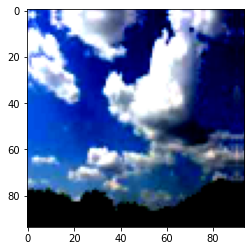In :
``show_example(*valid_ds)``
```Clipping input data to the valid range for imshow with RGB data ([0..1] for floats or [0..255] for integers). ```
```Label: sunrise (3) ```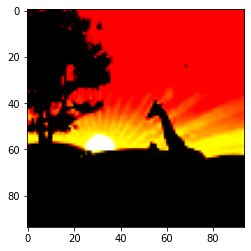Next, we can create data loaders for retrieving images in batches. We'll use a batch size of 32.

In :
``batch_size = 32``
In :
``````# PyTorch data loaders
train_dl = DataLoader(train_ds, batch_size, shuffle=True, num_workers=3, pin_memory=True)
valid_dl = DataLoader(valid_ds, batch_size, num_workers=3, pin_memory=True)``````

Let's take a look at some sample images from the training dataloader. To display the images, we'll need to denormalize the pixels values to bring them back into the range (0,1).

In :
``````def denormalize(images, means, stds):
means = torch.tensor(means).reshape(1, 3, 1, 1)
stds = torch.tensor(stds).reshape(1, 3, 1, 1)
return images * stds + means

def show_batch(dl):
for images, labels in dl:
fig, ax = plt.subplots(figsize=(12, 12))
ax.set_xticks([]); ax.set_yticks([])
denorm_images = denormalize(images, *stats)
ax.imshow(make_grid(denorm_images[:32], nrow=8).permute(1, 2, 0).clamp(0,1))
break``````
In :
``show_batch(train_dl)``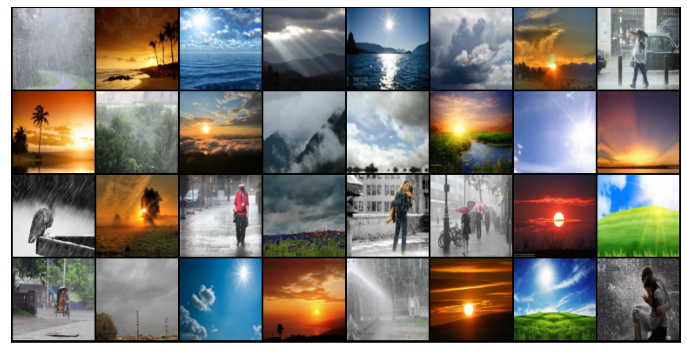### Using a GPU

GPU boasts of superior parallel computation power than CPU and is highly used when doing classificaton problems to train models faster. To seamlessly use a GPU, if one is available, we define a couple of helper functions (`get_default_device` & `to_device`) and a helper class DeviceDataLoader to move our model & data to the GPU as required.

In :
``````def get_default_device():
"""Pick GPU if available, else CPU"""
if torch.cuda.is_available():
else:

def to_device(data, device):
"""Move tensor(s) to chosen device"""
if isinstance(data, (list,tuple)):
return [to_device(x, device) for x in data]
return data.to(device, non_blocking=True)

"""Wrap a dataloader to move data to a device"""
def __init__(self, dl, device):
self.dl = dl
self.device = device

def __iter__(self):
"""Yield a batch of data after moving it to device"""
for b in self.dl:
yield to_device(b, self.device)

def __len__(self):
"""Number of batches"""
return len(self.dl)``````
In :
``````# Let's check whether we are using a CPU or GPU
device = get_default_device()
device
``````
Out:
``device(type='cuda')``

Great! We can see GPU is enabled and so we can now wrap our training and validation data loaders using `DeviceDataLoader` for automatically transferring batches of data to the GPU.

In :
``````train_dl = DeviceDataLoader(train_dl, device)
``````

#### Let's save our work.

In [ ]:
``!pip install jovian --upgrade --quiet``
In [ ]:
``import jovian``
In [ ]:
``jovian.commit(project=project_name, environment=None)``

## Model Creation using ResNet9 Architecture

We will be using CNNs with the addition of the resudial block, which adds the original input back to the output feature map obtained by passing the input through one or more convolutional layers. This seeming small change produces a drastic improvement in the performance of the model. Also, after each convolutional layer, we'll add a batch normalization layer, which normalizes the outputs of the previous layer.

We will be using ResNet9 containing 8 convolutional layers, 8 ReLU layers, 3 MaxPool layers, 1 Flatten layer and 1 Dropout Layer and all this can also avoid some unwanted phenomenon like overfitting.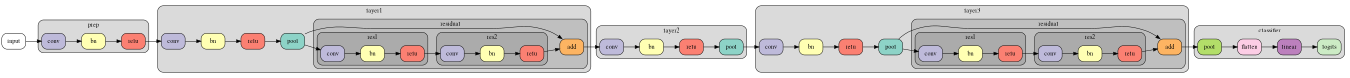In :
``````def accuracy(outputs, labels):
_, preds = torch.max(outputs, dim=1)

class ImageClassificationBase(nn.Module):
def training_step(self, batch):
images, labels = batch
out = self(images)                  # Generate predictions
loss = F.cross_entropy(out, labels) # Calculate loss
return loss

def validation_step(self, batch):
images, labels = batch
out = self(images)                    # Generate predictions
loss = F.cross_entropy(out, labels)   # Calculate loss
acc = accuracy(out, labels)           # Calculate accuracy
return {'val_loss': loss.detach(), 'val_acc': acc}

def validation_epoch_end(self, outputs):
batch_losses = [x['val_loss'] for x in outputs]
epoch_loss = torch.stack(batch_losses).mean()   # Combine losses
batch_accs = [x['val_acc'] for x in outputs]
epoch_acc = torch.stack(batch_accs).mean()      # Combine accuracies
return {'val_loss': epoch_loss.item(), 'val_acc': epoch_acc.item()}

def epoch_end(self, epoch, result):
print("Epoch [{}], last_lr: {:.5f}, train_loss: {:.4f}, val_loss: {:.4f}, val_acc: {:.4f}".format(
epoch, result['lrs'][-1], result['train_loss'], result['val_loss'], result['val_acc']))``````
In :
``````def conv_block(in_channels, out_channels, pool=False):
layers = [nn.Conv2d(in_channels, out_channels, kernel_size=3, padding=1),
nn.BatchNorm2d(out_channels),
nn.ReLU(inplace=True)]
if pool: layers.append(nn.MaxPool2d(2))
return nn.Sequential(*layers)

class ResNet9(ImageClassificationBase):
def __init__(self, in_channels, num_classes):
super().__init__()

self.conv1 = conv_block(in_channels, 64)
self.conv2 = conv_block(64, 128, pool=True)
self.res1 = nn.Sequential(conv_block(128, 128), conv_block(128, 128))

self.conv3 = conv_block(128, 256, pool=True)
self.conv4 = conv_block(256, 512, pool=True)
self.res2 = nn.Sequential(conv_block(512, 512), conv_block(512, 512))

self.classifier = nn.Sequential(nn.MaxPool2d(11),
nn.Flatten(),
nn.Dropout(0.2),
nn.Linear(512, num_classes))
def forward(self, xb):
out = self.conv1(xb)
out = self.conv2(out)
out = self.res1(out) + out
out = self.conv3(out)
out = self.conv4(out)
out = self.res2(out) + out
out = self.classifier(out)
return out``````
In :
``````model = to_device(ResNet9(3, 4), device)
model
``````
Out:
``````ResNet9(
(conv1): Sequential(
(0): Conv2d(3, 64, kernel_size=(3, 3), stride=(1, 1), padding=(1, 1))
(1): BatchNorm2d(64, eps=1e-05, momentum=0.1, affine=True, track_running_stats=True)
(2): ReLU(inplace=True)
)
(conv2): Sequential(
(0): Conv2d(64, 128, kernel_size=(3, 3), stride=(1, 1), padding=(1, 1))
(1): BatchNorm2d(128, eps=1e-05, momentum=0.1, affine=True, track_running_stats=True)
(2): ReLU(inplace=True)
(3): MaxPool2d(kernel_size=2, stride=2, padding=0, dilation=1, ceil_mode=False)
)
(res1): Sequential(
(0): Sequential(
(0): Conv2d(128, 128, kernel_size=(3, 3), stride=(1, 1), padding=(1, 1))
(1): BatchNorm2d(128, eps=1e-05, momentum=0.1, affine=True, track_running_stats=True)
(2): ReLU(inplace=True)
)
(1): Sequential(
(0): Conv2d(128, 128, kernel_size=(3, 3), stride=(1, 1), padding=(1, 1))
(1): BatchNorm2d(128, eps=1e-05, momentum=0.1, affine=True, track_running_stats=True)
(2): ReLU(inplace=True)
)
)
(conv3): Sequential(
(0): Conv2d(128, 256, kernel_size=(3, 3), stride=(1, 1), padding=(1, 1))
(1): BatchNorm2d(256, eps=1e-05, momentum=0.1, affine=True, track_running_stats=True)
(2): ReLU(inplace=True)
(3): MaxPool2d(kernel_size=2, stride=2, padding=0, dilation=1, ceil_mode=False)
)
(conv4): Sequential(
(0): Conv2d(256, 512, kernel_size=(3, 3), stride=(1, 1), padding=(1, 1))
(1): BatchNorm2d(512, eps=1e-05, momentum=0.1, affine=True, track_running_stats=True)
(2): ReLU(inplace=True)
(3): MaxPool2d(kernel_size=2, stride=2, padding=0, dilation=1, ceil_mode=False)
)
(res2): Sequential(
(0): Sequential(
(0): Conv2d(512, 512, kernel_size=(3, 3), stride=(1, 1), padding=(1, 1))
(1): BatchNorm2d(512, eps=1e-05, momentum=0.1, affine=True, track_running_stats=True)
(2): ReLU(inplace=True)
)
(1): Sequential(
(0): Conv2d(512, 512, kernel_size=(3, 3), stride=(1, 1), padding=(1, 1))
(1): BatchNorm2d(512, eps=1e-05, momentum=0.1, affine=True, track_running_stats=True)
(2): ReLU(inplace=True)
)
)
(classifier): Sequential(
(0): MaxPool2d(kernel_size=11, stride=11, padding=0, dilation=1, ceil_mode=False)
(1): Flatten(start_dim=1, end_dim=-1)
(2): Dropout(p=0.2, inplace=False)
(3): Linear(in_features=512, out_features=4, bias=True)
)
)``````

## Training the model

Before we train the model, we're going to make a bunch of small but important improvements to our fit function:

• Learning rate scheduling: Instead of using a fixed learning rate, we will use a learning rate scheduler, which will change the learning rate after every batch of training. There are many strategies for varying the learning rate during training, and the one we'll use is called the One Cycle Learning Rate Policy, which involves starting with a low learning rate, gradually increasing it batch-by-batch to a high learning rate for about 30% of epochs, then gradually decreasing it to a very low value for the remaining epochs.

• Weight decay: We also use weight decay, which is yet another regularization technique which prevents the weights from becoming too large by adding an additional term to the loss function.

• Gradient clipping: Apart from the layer weights and outputs, it is also helpful to limit the values of gradients to a small range to prevent undesirable changes in parameters due to large gradient values. This simple yet effective technique is called gradient clipping.

Let's define a fit_one_cycle function to incorporate these changes. We'll also record the learning rate used for each batch.

In :
``````@torch.no_grad()
model.eval()
outputs = [model.validation_step(batch) for batch in val_loader]
return model.validation_epoch_end(outputs)

def get_lr(optimizer):
for param_group in optimizer.param_groups:
return param_group['lr']

torch.cuda.empty_cache()
history = []

# Set up cutom optimizer with weight decay
optimizer = opt_func(model.parameters(), max_lr, weight_decay=weight_decay)
# Set up one-cycle learning rate scheduler
sched = torch.optim.lr_scheduler.OneCycleLR(optimizer, max_lr, epochs=epochs,

for epoch in range(epochs):
# Training Phase
model.train()
train_losses = []
lrs = []
loss = model.training_step(batch)
train_losses.append(loss)
loss.backward()

optimizer.step()

# Record & update learning rate
lrs.append(get_lr(optimizer))
sched.step()

# Validation phase
result['train_loss'] = torch.stack(train_losses).mean().item()
result['lrs'] = lrs
model.epoch_end(epoch, result)
history.append(result)
return history``````
In :
``````history = [evaluate(model,valid_dl)]
history
``````
Out:
``[{'val_acc': 0.3828125, 'val_loss': 1.3828785419464111}]``

We're now ready to train our model. We'll be using the Adam optimizer which uses techniques like momentum and adaptive learning rates for faster training.

In :
``````epochs = 15
max_lr = 0.01
weight_decay = 1e-4
``````
In :
``````%%time
history += fit_one_cycle(epochs, max_lr, model, train_dl, valid_dl,
weight_decay=weight_decay,
opt_func=opt_func)
``````
```Epoch , last_lr: 0.00147, train_loss: 0.6951, val_loss: 1.5184, val_acc: 0.7656 Epoch , last_lr: 0.00431, train_loss: 0.4704, val_loss: 1.3232, val_acc: 0.8359 Epoch , last_lr: 0.00757, train_loss: 0.7740, val_loss: 1.7710, val_acc: 0.7188 Epoch , last_lr: 0.00971, train_loss: 1.3603, val_loss: 14.7880, val_acc: 0.3438 Epoch , last_lr: 0.00994, train_loss: 0.9536, val_loss: 1.0892, val_acc: 0.7500 Epoch , last_lr: 0.00950, train_loss: 0.5475, val_loss: 0.6270, val_acc: 0.8047 Epoch , last_lr: 0.00867, train_loss: 0.9185, val_loss: 0.3355, val_acc: 0.8828 Epoch , last_lr: 0.00750, train_loss: 1.0150, val_loss: 0.6012, val_acc: 0.8750 Epoch , last_lr: 0.00611, train_loss: 1.0372, val_loss: 0.5745, val_acc: 0.8281 Epoch , last_lr: 0.00463, train_loss: 0.4931, val_loss: 0.5226, val_acc: 0.8828 Epoch , last_lr: 0.00317, train_loss: 0.5438, val_loss: 0.4737, val_acc: 0.9062 Epoch , last_lr: 0.00188, train_loss: 0.6323, val_loss: 0.4547, val_acc: 0.8594 Epoch , last_lr: 0.00087, train_loss: 0.4653, val_loss: 0.3260, val_acc: 0.9141 Epoch , last_lr: 0.00022, train_loss: 0.5564, val_loss: 0.3657, val_acc: 0.9062 Epoch , last_lr: 0.00000, train_loss: 0.2120, val_loss: 0.2915, val_acc: 0.9375 CPU times: user 18.2 s, sys: 10.8 s, total: 29 s Wall time: 2min 15s ```
In :
``train_time='2:15'``

Our model trained to over 93% accuracy after 15 epochs in under 3 minutes!

Let's plot the valdation set accuracies to study how the model improves over time.

In :
``````def plot_accuracies(history):
accuracies = [x['val_acc'] for x in history]
plt.plot(accuracies, '-x')
plt.xlabel('epoch')
plt.ylabel('accuracy')
plt.title('Accuracy vs. No. of epochs');``````
In :
``plot_accuracies(history)``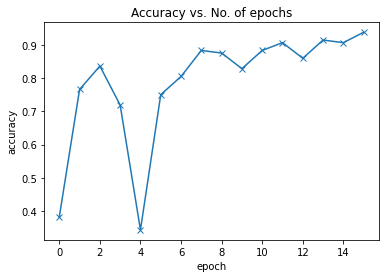We can also plot the training and validation losses to study the trend.

In :
``````def plot_losses(history):
train_losses = [x.get('train_loss') for x in history]
val_losses = [x['val_loss'] for x in history]
plt.plot(train_losses, '-bx')
plt.plot(val_losses, '-rx')
plt.xlabel('epoch')
plt.ylabel('loss')
plt.legend(['Training', 'Validation'])
plt.title('Loss vs. No. of epochs');``````
In :
``plot_losses(history)``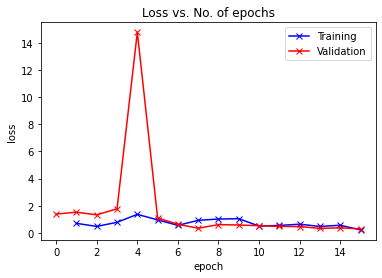Finally, let's visualize how the learning rate changed over time, batch-by-batch over all the epochs.

In :
``````def plot_lrs(history):
lrs = np.concatenate([x.get('lrs', []) for x in history])
plt.plot(lrs)
plt.xlabel('Batch no.')
plt.ylabel('Learning rate')
plt.title('Learning Rate vs. Batch no.');``````
In :
``plot_lrs(history)``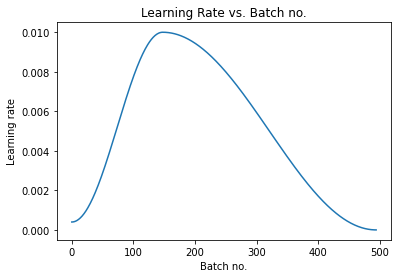As expected, the learning rate starts at a low value, and gradually increases for 30% of the iterations to a maximum value of 0.01, and then gradually decreases to a very small value.

## Testing with individual images

Now it's time to test out our model with some images from the predefined test dataset of 100 images.

In :
``````def predict_image(img, model):
# Convert to a batch of 1
xb = to_device(img.unsqueeze(0), device)
# Get predictions from model
yb = model(xb)
# Pick index with highest probability
_, preds  = torch.max(yb, dim=1)
# Retrieve the class label
return train_ds.classes[preds.item()]``````
In :
``````img, label = valid_ds
plt.imshow(img.permute(1, 2, 0).clamp(0, 1))
print('Label:', train_ds.classes[label], ', Predicted:', predict_image(img, model))``````
```Label: cloudy , Predicted: cloudy ```